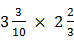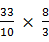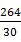### Sample Problem

Multiply. Simplify your answer and write it as a proper fraction or as a whole or mixed number.=

#### Solution

Step 1: Write whole numbers as improper fractions

Step 2: Write mixed numbers as improper fractions.

Step 3: Multiply fractions.

Step 4: Reduce to simplest form=====